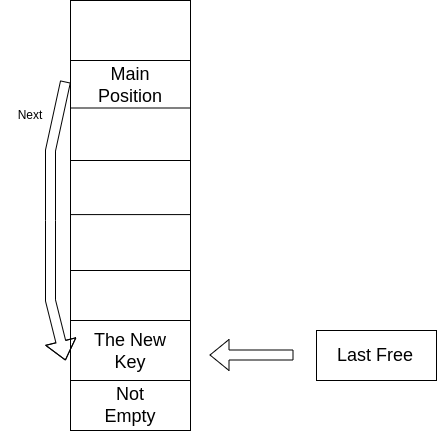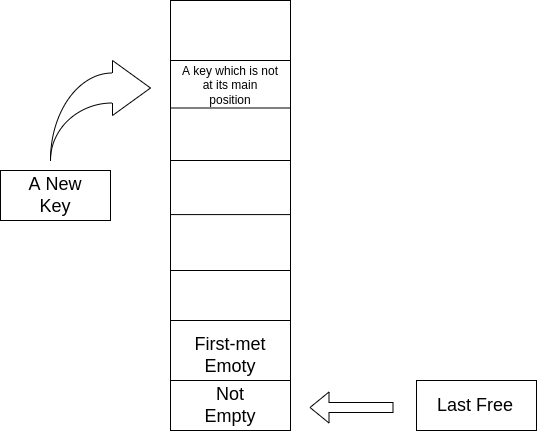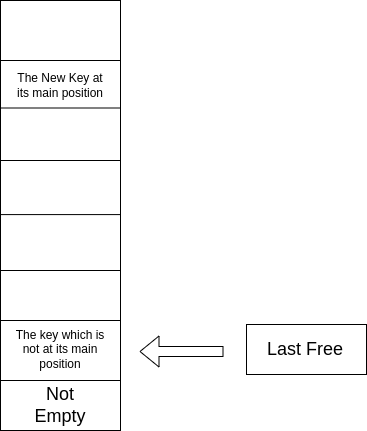## Lua Table 简介#

• 数组是键值为正整数的表；

• 由于键值也可以是其他类型，故表也可以实现字典这种数据结构；

• 由于函数也是 Lua 的第一公民，所以成员函数也可以通过表来实现；

• 模块和包之间的从属关系也是通过 table 来表示的。

Lua 中的 table 使用十分广泛，这也导致了 Lua 使用起来有着 纯真的、野性的 简洁的美。

## Lua 解释器中的哈希函数#

``````unsigned int luaS_hash (const char *str, size_t l, unsigned int seed) {
unsigned int h = seed ^ cast_uint(l);
for (; l > 0; l--)
h ^= ((h<<5) + (h>>2) + cast_byte(str[l - 1]));
return h;
}
``````

``````unsigned int luaS_hash(struct lua_State* L, const char* str, unsigned int l, unsigned int h) {
h = h ^ l;
unsigned int step = (l >> 5) + 1;
for (int i = 0; i < l; i = i + step) {
h ^= (h << 5) + (h >> 2) + cast(lu_byte, str[i]);
}
return h;
}
``````

``````index = hash_value & ((1U << lsizenode) - 1)
``````

## Lua 解释器中的重要类型#

``````/*
** Union of all Lua values
*/
typedef union Value {
struct GCObject *gc;    /* collectable objects */
void *p;         /* light userdata */
lua_CFunction f; /* light C functions */
lua_Integer i;   /* integer numbers */
lua_Number n;    /* float numbers */
} Value;
``````

``````#define TValuefields	Value value_; lu_byte tt_

typedef struct TValue {
TValuefields;
} TValue;
``````

TValue 长度为 12 个字节，可以使用 NaN Trick 节约空间。

``````typedef union Node {
struct NodeKey {
TValuefields;  /* fields for value */
lu_byte key_tt;  /* key type */
int next;  /* for chaining */
Value key_val;  /* key value */
} u;
TValue i_val;  /* direct access to node's value as a proper 'TValue' */
} Node;
``````

``````typedef struct Table {
lu_byte flags;  /* 1<<p means tagmethod(p) is not present */
lu_byte lsizenode;  /* log2 of size of 'node' array */
unsigned int alimit;  /* "limit" of 'array' array */
TValue *array;  /* array part */
Node *node;
Node *lastfree;  /* any free position is before this position */
struct Table *metatable;
GCObject *gclist;
} Table;
``````

## Table 相关操作#

### get 操作#

``````const TValue *luaH_getint (Table *t, lua_Integer key) {
if (l_castS2U(key) - 1u < t->alimit)  /* 'key' in [1, t->alimit]? */
return &t->array[key - 1];
else if (!limitequalsasize(t) &&  /* key still may be in the array part? */
(l_castS2U(key) == t->alimit + 1 ||
l_castS2U(key) - 1u < luaH_realasize(t))) {
t->alimit = cast_uint(key);  /* probably '#t' is here now */
return &t->array[key - 1];
}
else {
Node *n = hashint(t, key);
for (;;) {  /* check whether 'key' is somewhere in the chain */
if (keyisinteger(n) && keyival(n) == key)
return gval(n);  /* that's it */
else {
int nx = gnext(n);
if (nx == 0) break;
n += nx;
}
}
return &absentkey;
}
}
``````

### set 操作#

``````void luaH_setint (lua_State *L, Table *t, lua_Integer key, TValue *value) {
const TValue *p = luaH_getint(t, key);
if (isabstkey(p)) {
TValue k;
setivalue(&k, key);
luaH_newkey(L, t, &k, value);
}
else
setobj2t(L, cast(TValue *, p), value);
}
``````

luaH_newkey 是一个很重要的函数，它的主要逻辑和函数原型如下：

``````/*
** inserts a new key into a hash table; first, check whether key's main
** position is free. If not, check whether colliding node is in its main
** position or not: if it is not, move colliding node to an empty place and
** put new key in its main position; otherwise (colliding node is in its main
** position), new key goes to an empty position.
*/
void luaH_newkey (lua_State *L, Table *t, const TValue *key, TValue *value);
``````

• 冲突节点在自己主位置的情况：• 冲突节点在自己主位置的情况：lastfree 的移动：初始化时指向 node 数组的最后一位。在这之后，一般情况下只会使用自减操作向左移动。

## resize 操作#

``````/*
** Count keys in array part of table 't': Fill 'nums[i]' with
** number of keys that will go into corresponding slice and return
** total number of non-nil keys.
*/
static unsigned int numusearray (const Table *t, unsigned int *nums) {
int lg;
unsigned int ttlg;  /* 2^lg */
unsigned int ause = 0;  /* summation of 'nums' */
unsigned int i = 1;  /* count to traverse all array keys */
unsigned int asize = limitasasize(t);  /* real array size */
/* traverse each slice */
for (lg = 0, ttlg = 1; lg <= MAXABITS; lg++, ttlg *= 2) {
unsigned int lc = 0;  /* counter */
unsigned int lim = ttlg;
if (lim > asize) {
lim = asize;  /* adjust upper limit */
if (i > lim)
break;  /* no more elements to count */
}
/* count elements in range (2^(lg - 1), 2^lg] */
for (; i <= lim; i++) {
if (!isempty(&t->array[i-1]))
lc++;
}
nums[lg] += lc;
ause += lc;
}
return ause;
}
``````

``````static unsigned int computesizes (unsigned int nums[], unsigned int *pna) {
int i;
unsigned int twotoi;  /* 2^i (candidate for optimal size) */
unsigned int a = 0;  /* number of elements smaller than 2^i */
unsigned int na = 0;  /* number of elements to go to array part */
unsigned int optimal = 0;  /* optimal size for array part */
/* loop while keys can fill more than half of total size */
for (i = 0, twotoi = 1;
twotoi > 0 && *pna > twotoi / 2;
i++, twotoi *= 2) {
a += nums[i];
if (a > twotoi/2) {  /* more than half elements present? */
optimal = twotoi;  /* optimal size (till now) */
na = a;  /* all elements up to 'optimal' will go to array part */
}
}
lua_assert((optimal == 0 || optimal / 2 < na) && na <= optimal);
*pna = na;
return optimal;
}
``````

``````/*
** nums[i] = number of keys 'k' where 2^(i - 1) < k <= 2^i
*/
static void rehash (lua_State *L, Table *t, const TValue *ek) {
unsigned int asize;  /* optimal size for array part */
unsigned int na;  /* number of keys in the array part */
unsigned int nums[MAXABITS + 1];
int i;
int totaluse;
for (i = 0; i <= MAXABITS; i++) nums[i] = 0;  /* reset counts */
setlimittosize(t);
na = numusearray(t, nums);  /* count keys in array part */
totaluse = na;  /* all those keys are integer keys */
totaluse += numusehash(t, nums, &na);  /* count keys in hash part */
/* count extra key */
if (ttisinteger(ek))
na += countint(ivalue(ek), nums);
totaluse++;
/* compute new size for array part */
asize = computesizes(nums, &na);
/* resize the table to new computed sizes */
luaH_resize(L, t, asize, totaluse - na);
}
``````

## next 操作#

``````int luaH_next (lua_State *L, Table *t, StkId key) {
unsigned int asize = luaH_realasize(t);
unsigned int i = findindex(L, t, s2v(key), asize);  /* find original key */
for (; i < asize; i++) {  /* try first array part */
if (!isempty(&t->array[i])) {  /* a non-empty entry? */
setivalue(s2v(key), i + 1);
setobj2s(L, key + 1, &t->array[i]);
return 1;
}
}
for (i -= asize; cast_int(i) < sizenode(t); i++) {  /* hash part */
if (!isempty(gval(gnode(t, i)))) {  /* a non-empty entry? */
Node *n = gnode(t, i);
getnodekey(L, s2v(key), n);
setobj2s(L, key + 1, gval(n));
return 1;
}
}
return 0;  /* no more elements */
}
``````